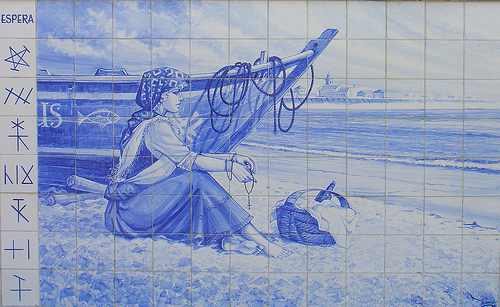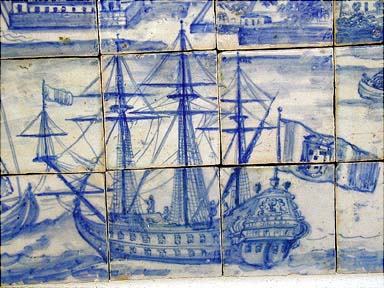What is Universal Logic? In the same way that universal algebra is a general theory of algebraic structures, universal logic is a general theory of logical structures. During the 20th century, numerous logics have been created: intuitionistic logic, deontic logic, many-valued logic, relevant logic, linear logic, non monotonic logic, etc. Universal logic is not a new logic, it is a way of unifying this multiplicity of logics by developing general tools and concepts that can be applied to all logics.  PROGRAM One aim of universal logic is to determine the domain of validity of such and such metatheorem (e.g. the completeness theorem) and to give general formulations of metatheorems. This is very useful for applications and helps to make the distinction between what is really essential to a particular logic and what is not, and thus gives a better understanding of this particular logic. Universal logic can also be seen as a toolkit for producing a specific logic required for a given situation, e.g. a paraconsistent deontic temporal logic.Universal logic helps to clarify basic concepts explaining what is an extension and what is a deviation of a given logic, what does it mean for a logic to be equivalent or translatable into another one. It allows to give precise definitions of notions often discussed by philosophers: truth-functionality, extensionality, logical form, etc. Aim of the Event This is the third edition of a world event dedicated to universal logic. This event is a combination of a school and a congress. The school offers 21 tutorials on a wide range of subjects. The congress will follow with invited talks and contributed talks organized in many sessions including 10 special sessions. There will also be a contest.This event is intended to be a major event in logic, providing a platform for future research guidelines. Such an event is of interest for all people dealing with logic in one way or another: pure logicians, mathematicians, computer scientists, AI researchers, linguists, psychologists, philosophers, etc. The whole event will happen nearby Lisbon, Portugal, a place which was the departure point of many adventures. UNILOG'2010 Join this adventure! <>
>